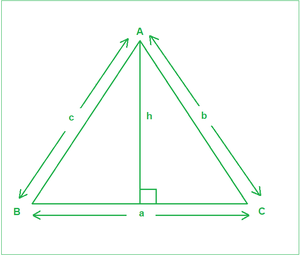GeeksforGeeks App
Open AppBrowser
Continue

# Sine Rule with Derivation, Example and Implementation

Given angles(in degrees) A, C, and the side c, corresponding to the figure below, the task is to find the remaining two sides a and b.Examples:

Input: A = 45, C = 35, c = 23
Output:
28.35
39.49
Explanation:
a is 28.35 and b is 39.49

Input: A = 45, C = 45, c = 10
Output:
10
14.14

Approach: The idea is to use Sine rule. It states that the sides of any triangle are proportional to the sine of the angles opposite to them. a / Sin(A) = b / Sin(B) = c / Sin(C). The derivation is described below:

As is evident from the figure above:

A perpendicular of length h has been drawn on BC from A. From General trigonometric rules:

SinB=h/c——–(1)

SinC=h/b——–(2)

From the above two equations, we get:

c x SinB=b x SinC

Or b/SinB=c/SinC—–(3)

Similarly, if a perpendicular is drawn from B to AC, we can get:

a/SinA=c/SinC——-(4)

From Equations (3) and (4), we get:

a/SinA=b/SinB=c/SinC

Follow the steps below to solve the problem:

• Change the angles A and C from degrees to radians to be able to be used in the inbuilt functions.
• Calculate the angle B using the observation that sums of angles of a triangle sums up to 180 degrees.
• Use the Sine rule to calculate the sides a and b.

Below is the implementation of the above approach:

## C++14

 `// C++ program for the above approach``#include ``using` `namespace` `std;` `// Function to calculate remaining two sides``void` `findSides(``double` `A, ``double` `C, ``double` `c)``{``    ``// Calculate angle B``    ``double` `B = 180 - (A + C);` `    ``// Convert angles to their respective radians for``    ``// using trigonometric functions``    ``A = A * (3.14159 / 180);``    ``C = C * (3.14159 / 180);``    ``B = B * (3.14159 / 180);` `    ``// Sine rule``    ``double` `a = (c / ``sin``(C)) * ``sin``(A);``    ``double` `b = (c / ``sin``(C)) * ``sin``(B);` `    ``// Precision of 2 decimal spaces``    ``cout << fixed << setprecision(2);` `    ``// Print the answer``    ``cout << a << endl;``    ``cout << b << endl;``}` `// Driver Code``int` `main()``{``    ``// Input``    ``double` `A = 45.0;``    ``double` `C = 35.0;``    ``double` `c = 23;` `    ``// Function Call``    ``findSides(A, C, c);``    ``return` `0;``}`

## Java

 `// Java program for the above approach``class` `GFG{` `// Function to calculate remaining two sides``static` `void` `findSides(``double` `A, ``double` `C,``                      ``double` `c)``{``    ` `    ``// Calculate angle B``    ``double` `B = ``180` `- (A + C);` `    ``// Convert angles to their respective``    ``// radians for using trigonometric functions``    ``A = A * (``3.14159` `/ ``180``);``    ``C = C * (``3.14159` `/ ``180``);``    ``B = B * (``3.14159` `/ ``180``);` `    ``// Sine rule``    ``double` `a = (c / Math.sin(C)) * Math.sin(A);``    ``double` `b = (c / Math.sin(C)) * Math.sin(B);` `    ``// Print the answer``    ``System.out.println(String.format(``"%.2f"``, a));``    ``System.out.println(String.format(``"%.2f"``, b));``}` `// Driver code``public` `static` `void` `main(String[] args)``{``    ` `    ``// Input``    ``double` `A = ``45.0``;``    ``double` `C = ``35.0``;``    ``double` `c = ``23``;` `    ``// Function Call``    ``findSides(A, C, c);``}``}` `// This code is contributed by abhinavjain194`

## Python3

 `# Python3 program for the above approach``import` `math` `# Function to calculate remaining two sides``def` `findSides(A, C, c):``    ` `    ``# Calculate angle B``    ``B ``=` `180` `-` `(A ``+` `C)` `    ``# Convert angles to their respective radians``    ``# for using trigonometric functions``    ``A ``=` `A ``*` `(``3.14159` `/` `180``)``    ``C ``=` `C ``*` `(``3.14159` `/` `180``)``    ``B ``=` `B ``*` `(``3.14159` `/` `180``)` `    ``# Sine rule``    ``a ``=` `(c ``/` `math.sin(C)) ``*` `math.sin(A)``    ``b ``=` `(c ``/` `math.sin(C)) ``*` `math.sin(B)` `    ``# Precision of 2 decimal spaces` `    ``# Print the answer``    ``print``(``"{0:.2f}"``.``format``(a))``    ``print``(``"{0:.2f}"``.``format``(b))` `# Driver Code` `# Input``A ``=` `45.0``C ``=` `35.0``c ``=` `23` `# Function Call``findSides(A, C, c)` `# This code is contributed by target_2`

## C#

 `// C# program for the above approach``using` `System;``class` `GFG{` `// Function to calculate remaining two sides``static` `void` `findSides(``double` `A, ``double` `C,``                      ``double` `c)``{``    ` `    ``// Calculate angle B``    ``double` `B = 180 - (A + C);` `    ``// Convert angles to their respective``    ``// radians for using trigonometric functions``    ``A = A * (3.14159 / 180);``    ``C = C * (3.14159 / 180);``    ``B = B * (3.14159 / 180);` `    ``// Sine rule``    ``double` `a = (c / Math.Sin(C)) * Math.Sin(A);``    ``double` `b = (c / Math.Sin(C)) * Math.Sin(B);` `    ``// Print the answer``    ``Console.WriteLine(``"{0:F2}"``,a);``    ``Console.WriteLine(``"{0:F2}"``,b);``}` `// Driver code``public` `static` `void` `Main(String[] args)``{``    ` `    ``// Input``    ``double` `A = 45.0;``    ``double` `C = 35.0;``    ``double` `c = 23;` `    ``// Function Call``    ``findSides(A, C, c);``}``}` `// This code is contributed by shivanisinghss2110`

## Javascript

 ``

Output

```28.35
39.49```

Time Complexity: O(1)
Auxiliary Space: O(1)

My Personal Notes arrow_drop_up